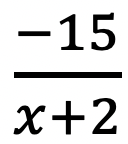# Dividing Polynomials: Algebra 2/Trig.

Greeting math peeps! In this post we are going to go over dividing polynomials! At some point, you may need to know how to answer these types of questions. The cool thing about dividing polynomials is that it’s the same long division you did way back in grade school (except now with a lot of x). Ok, let’s get to it and check out the question below:

Also, if you haven’t done so, check out the video related that corresponds to this problem on Youtube below! 🙂

Explanation:

How do I answer this question?

The question wants us to divide polynomials by using long division.

How do we do this?

Step 1: First we set up a good ole’ division problem with the divisor, dividend, and quotient to solve.

Step 2: Now we use long division like we used to back in the day! If you have any confusion about this please check out the video in this post.

## What if there’s a Remainder?

What happens when there is a remainder though!?!? When dividing polynomials with a remainder in the quotient, the answer is found and checked in a very similar way! Check it out in the example below:

Notice we represented the remainder by addingto our quotient! We just put the remainder over the divisor to represent this extra bit of solution.

Want more practice? Try solving these next few examples on your own.

## Solutions:

If you’re looking for more on dividing polynomials, check out this post on synthetic division and finding zeros here!

Still got questions? No problem! Don’t hesitate to comment with any questions or check out the video above. Happy calculating! 🙂

Posted on Categories Algebra 2/Trig, Uncategorized